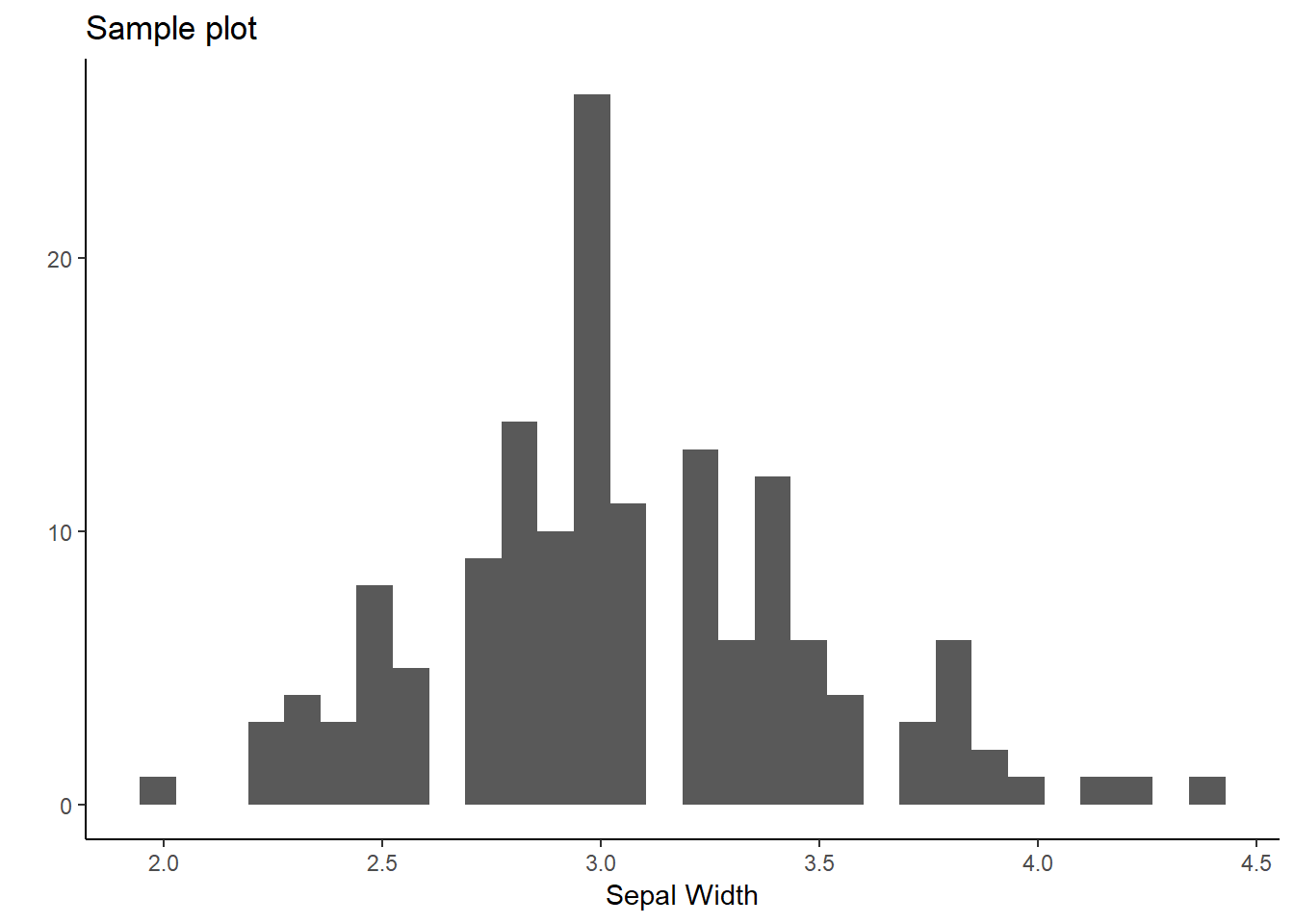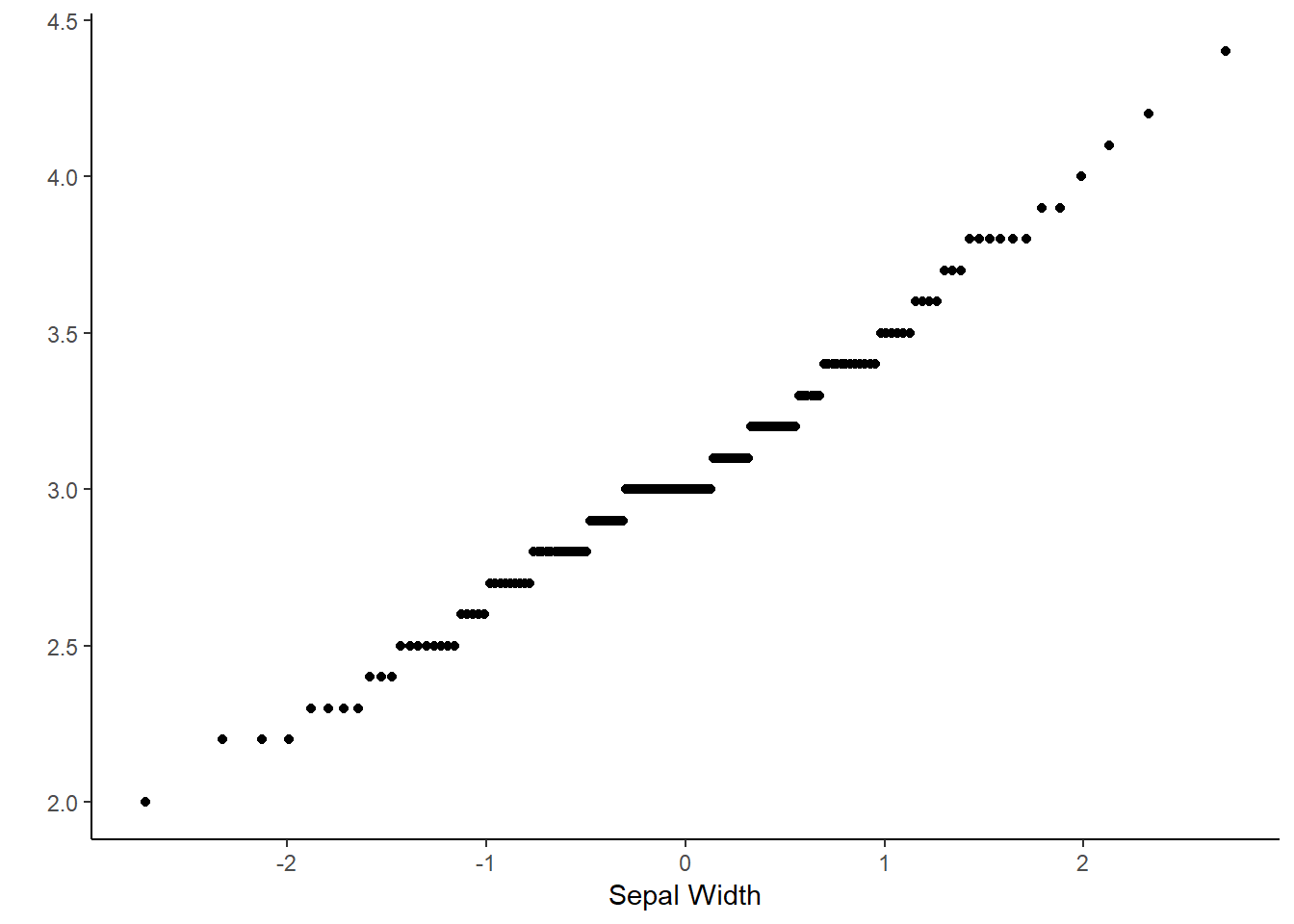# The Tableone package: Effiencent Table 1's for clinical studies

Every randomized study need a Table 1 to summarize the characteristics of the included participants. The tables consist of multiple calculations of means with standard deviation, medians with quartiles or summarizes of categorical variables. This can however be very time consuming to calculate if done by hard. Especially, because you often want to make several subgroups etc.

Some years ago I came across the R package tableone, which has now become my preffered tool for creating table 1’s.

Firstly, the package must be installed and loaded into a R session:

``````#Install "tableone", if not already installed and loaded:
if (!require("tableone")) install.packages("tableone"); library(tableone)
#I also like to use the tidyverse packages to plot and analyse the data:
if (!require("tidyverse")) install.packages("tidyverse"); library(tidyverse)

#Load some sample data, here the "iris" data set, or use your own data set:
data("iris")
attach(iris)``````

Take a look at the data set and determine how you would like to analyse the different variables. Use histograms, QQ-plots etc. to investigate the variables. The iris data consist of 5 different variables, four are continous (length and width) and one is a categorical (species).

``````a <- ggplot(iris, aes(iris\$Sepal.Width)) + geom_histogram() + theme_classic()
a + labs(x= "Sepal Width", y="", title = "Sample plot")````````ggplot(iris) + geom_qq(aes(sample = iris\$Sepal.Width)) + theme_classic() + labs( x = "Sepal Width", y="")````````#Choose which variables to include in table 1 (NB: All variables must be listed here)
variables <- c("Sepal.Width", "Sepal.Length", "Petal.Width")
#Which of the above chosen variables must be treated as a non-normal variable?
non_normal <- c()

#Use the argument strata for subgroups:
mytableone <- tableone::CreateTableOne( data = iris, vars = variables, strata = "Species")

#Adjust the amount of digits, use of test and more:
print(mytableone, nonnormal = non_normal, explain=TRUE, catDigits=1, contDigits=1, test=F)``````
``````##                           Stratified by Species
##                            setosa    versicolor virginica
##   n                         50        50         50
##   Sepal.Width (mean (sd))  3.4 (0.4) 2.8 (0.3)  3.0 (0.3)
##   Sepal.Length (mean (sd)) 5.0 (0.4) 5.9 (0.5)  6.6 (0.6)
##   Petal.Width (mean (sd))  0.2 (0.1) 1.3 (0.2)  2.0 (0.3)``````